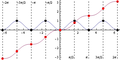File:Sinesquaredwithantiderivative.png

Sinesquaredwithantiderivative.png(360 × 181 pixels, file size: 9 KB, MIME type: image/png)

This file was created using Wolfram Mathematica 8.0 with the following code:

```f[x_] := Integrate[(Sin[t])^2, {t, 0, x}]
Plot[{(Sin[x])^2, f[x]}, {x, -2 Pi, 2 Pi}, AspectRatio -> 1/2,
Epilog -> {PointSize[0.02], Point[{0, 0}], Point[{Pi/2, 1}],
Point[{Pi, 0}], Point[{3 Pi/2, 1}], Point[{2 Pi, 0}],
Point[{-Pi/2, 1}], Point[{-Pi, 0}], Point[{-3 Pi/2, 1}],
Point[{-2 Pi, 0}], Red, Point[{0, 0}], Point[{Pi/2, Pi/4}],
Point[{Pi, Pi/2}], Point[{3 Pi/2, 3 Pi/4}], Point[{2 Pi, Pi}],
Point[{-Pi/2, -Pi/4}], Point[{-Pi, -Pi/2}],
Point[{-3 Pi/2, -3 Pi/4}], Point[{-2 Pi, -Pi}], Black, Dashed,
Line[{{Pi/2, -Pi}, {Pi/2, Pi}}], Line[{{Pi, -Pi}, {Pi, Pi}}],
Line[{{3 Pi/2, -Pi}, {3 Pi/2, Pi}}],
Line[{{2 Pi, -Pi}, {2 Pi, Pi}}], Line[{{-Pi/2, -Pi}, {-Pi/2, Pi}}],
Line[{{-Pi, -Pi}, {-Pi, Pi}}],
Line[{{-3 Pi/2, -Pi}, {-3 Pi/2, Pi}}],
Line[{{-2 Pi, -Pi}, {-2 Pi, Pi}}],
Text["\[Pi]/2", {Pi/2 - 0.3, -3}], Text["\[Pi]", {Pi - 0.2, -3}],
Text["3\[Pi]/2", {3 Pi/2 - 0.4, -3}],
Text["2\[Pi]", {2 Pi - 0.3, -3}],
Text["-\[Pi]/2", {-Pi/2 + 0.4, 3}], Text["-\[Pi]", {-Pi + 0.3, 3}],
Text["-3\[Pi]/2", {-3 Pi/2 + 0.5, 3}],
Text["-2\[Pi]", {-2 Pi + 0.4, 3}]}]```

File history

Click on a date/time to view the file as it appeared at that time.

Date/TimeThumbnailDimensionsUserComment
current16:47, 28 May 2012360 × 181 (9 KB)Vipul (talk | contribs)
00:52, 4 September 2011No thumbnail360 × 181 (9 KB)Vipul (talk | contribs)
20:21, 3 September 2011
Error creating thumbnail: File missing
360 × 181 (6 KB)Vipul (talk | contribs)This file was created using [http://www.wolfram.com/mathematica Wolfram Mathematica 8.0] with the following code: <pre>f[x_] := Integrate[(Sin[t])^2, {t, 0, x}] Plot[{(Sin[x])^2, f[x]}, {x, -2 Pi, 2 Pi}, AspectRatio -> 1/2]</pre>
• You cannot overwrite this file.

The following page links to this file: